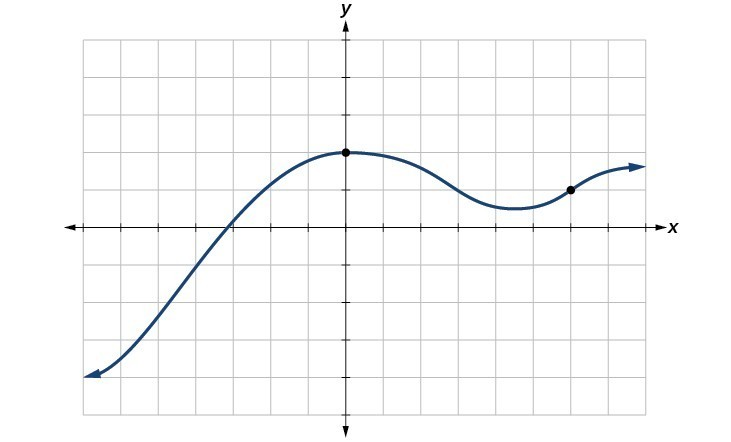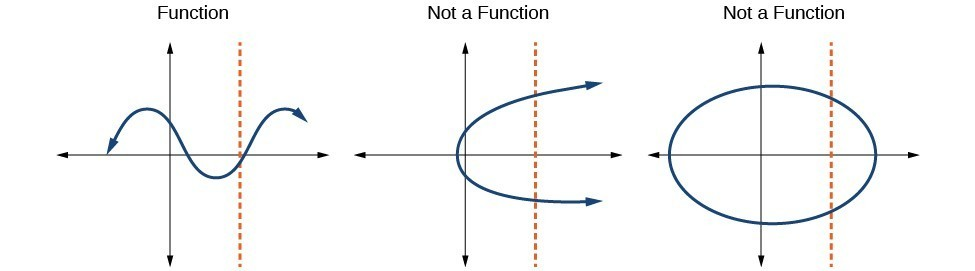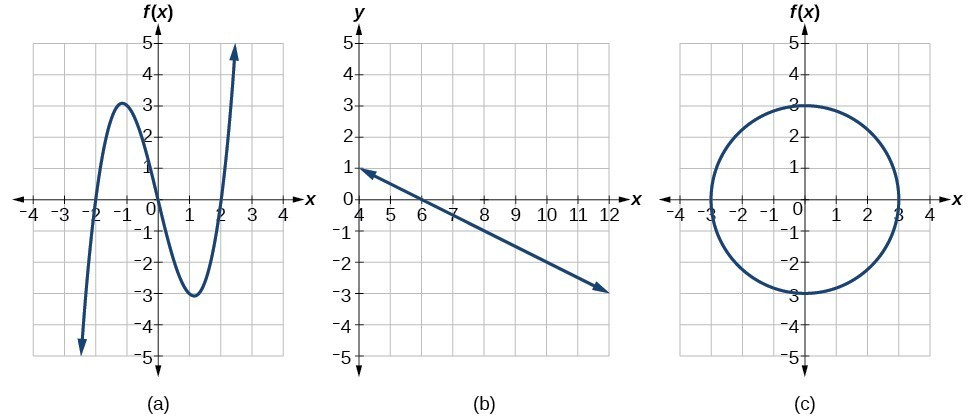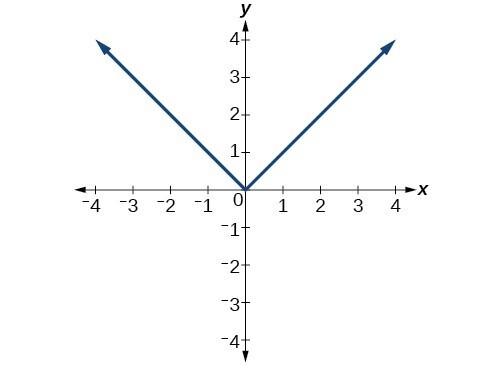## Use the vertical line test to identify functions

As we have seen in some examples above, we can represent a function using a graph. Graphs display a great many input-output pairs in a small space. The visual information they provide often makes relationships easier to understand. By convention, graphs are typically constructed with the input values along the horizontal axis and the output values along the vertical axis.

The most common graphs name the input value $x$ and the output value $y$, and we say $y$ is a function of $x$, or $y=f\left(x\right)$ when the function is named $f$. The graph of the function is the set of all points $\left(x,y\right)$ in the plane that satisfies the equation $y=f\left(x\right)$. If the function is defined for only a few input values, then the graph of the function is only a few points, where the x-coordinate of each point is an input value and the y-coordinate of each point is the corresponding output value. For example, the black dots on the graph in Figure 11 tell us that $f\left(0\right)=2$ and $f\left(6\right)=1$. However, the set of all points $\left(x,y\right)$ satisfying $y=f\left(x\right)$ is a curve. The curve shown includes $\left(0,2\right)$ and $\left(6,1\right)$ because the curve passes through those points.Figure 11

The vertical line test can be used to determine whether a graph represents a function. If we can draw any vertical line that intersects a graph more than once, then the graph does not define a function because a function has only one output value for each input value.Figure 12

### How To: Given a graph, use the vertical line test to determine if the graph represents a function.

1. Inspect the graph to see if any vertical line drawn would intersect the curve more than once.
2. If there is any such line, determine that the graph does not represent a function.

### Example 14: Applying the Vertical Line Test

Which of the graphs represent(s) a function $y=f\left(x\right)?$Figure 13

### Solution

If any vertical line intersects a graph more than once, the relation represented by the graph is not a function. Notice that any vertical line would pass through only one point of the two graphs shown in parts (a) and (b) of Figure 13. From this we can conclude that these two graphs represent functions. The third graph does not represent a function because, at most x-values, a vertical line would intersect the graph at more than one point.

### Try It 8

Does the graph in Figure 15 represent a function?Figure 15

Solution

## Using the Horizontal Line Test

Once we have determined that a graph defines a function, an easy way to determine if it is a one-to-one function is to use the horizontal line test. Draw horizontal lines through the graph. If any horizontal line intersects the graph more than once, then the graph does not represent a one-to-one function.

### How To: Given a graph of a function, use the horizontal line test to determine if the graph represents a one-to-one function.

1. Inspect the graph to see if any horizontal line drawn would intersect the curve more than once.
2. If there is any such line, determine that the function is not one-to-one.

### Example 15: Applying the Horizontal Line Test

Consider the functions (a), and (b)shown in the graphs in Figure 16.Figure 16

Are either of the functions one-to-one?

### Solution

The function in (a) is not one-to-one. The horizontal line shown in Figure 17 intersects the graph of the function at two points (and we can even find horizontal lines that intersect it at three points.)

The function in (b) is one-to-one. Any horizontal line will intersect a diagonal line at most once.